×#### Thank you for registering.

One of our academic counsellors will contact you within 1 working day.

Click to Chat

1800-1023-196

+91-120-4616500

CART 0

• 0

MY CART (5)

Use Coupon: CART20 and get 20% off on all online Study Material

ITEM
DETAILS
MRP
DISCOUNT
FINAL PRICE
Total Price: Rs.

There are no items in this cart.
Continue Shopping
```
the van der waals constants for a gas are:4 litre square atm molsquare inverse., b=.04 lit mol inverse .its boyle temperature is roughly-

```
11 years ago

```							Hi
In thermodynamics, the Boyle temperature is defined as the temperature for which the second virial coefficient, B2(T) vanishes, i.e. B2(T) = 0. Since higher order virial coefficients are generally much smaller than the second coefficient, the gas tends to behave as an ideal gas over a wider range of pressures when the temperature reaches the Boyle temperature. In any case, when the pressures are low, the second virial coefficient will be the only relevant one because the remaining concern terms of higher order on the pressure. We then have dZ / dp = 0 at p = 0, where Z is the compression factor.
So, if the options are available then one can determine that at which temp, the B(t) tends to zero.
```
11 years ago
```							Hi
At low pressures, all gases behave ideally, that is, they follow the Ideal Gas Law

pVm = RT

where p = pressure, Vm = molar volume, T = temperature and R is the Gas Constant.
However, at higher pressures gases deviate from the Ideal Gas Law, following a linear expansion of the form

pVm = RT{1 + B´p + C´p2 + ...}

sometimes stated as

pVm = RT{1 + B/Vm + C/Vm2 + ...}

The values of the virial coefficients B, C, ... depend on temperature, and many gases have a particular temperature at which B = B´ = 0. This temperature is called the Boyle Temperature, TB.
TB can be derived from the van der Waals equation of state for a real gas,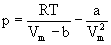In order to do that, we express the van der Waals equation as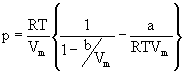So long as b/Vm < 1, we can expand the first term inside the brackets according to

(1 – x)-1 = 1 + x + x2 + ...

which gives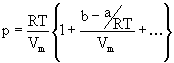Obviously the virial coefficient B is given by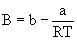At the Boyle Temperature B = 0, and so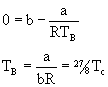(Tc = 8a/27Rb is the critical temperature of the gas. For a derivation of the relationship of the critical constants to the van der Waals constants, see P.W. Atkins, Physical Chemistry, 3d Ed. page 31.)

```
11 years ago
Think You Can Provide A Better Answer ?

## Other Related Questions on Physical Chemistry

View all Questions »### Course Features

• 731 Video Lectures
• Revision Notes
• Previous Year Papers
• Mind Map
• Study Planner
• NCERT Solutions
• Discussion Forum
• Test paper with Video Solution### Course Features

• 141 Video Lectures
• Revision Notes
• Test paper with Video Solution
• Mind Map
• Study Planner
• NCERT Solutions
• Discussion Forum
• Previous Year Exam Questions